# How to replace all characters before the first specific character

This post will guide you how to replace all characters before the first match of a specific character with a new text string in excel. How to replace substring before the first occurrence of the comma character with another substring in a text string using excel formula.

## Replace all characters before a specific character

If you want to replace characters before the first match of the comma character in a text string in Cell B1, you need to get all characters before the first comma firstly, and we have talked that how to extract text before a specific character in the previous post. So you still need to use the SUBSTITUTE function to replace the old_text with new_text. So you can use the following formula:

`=SUBSTITUTE(B1,LEFT(B1,FIND(",",B1,1)-1),"excelhow",1)`

Let’s see how this formula works:

=FIND(“,”,B1)-1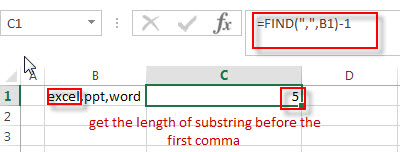This formula returns the position of the first match of the comma character in a text string in Cell B1, then subtract 1 by the position number to get the length of substring before the first comma, so it returns 5. And the returned value goes into the LEFT function as its num_chars argument.

=LEFT(B1,FIND(“,”,B1)-1)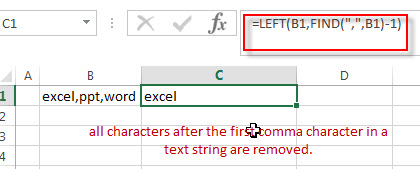This formula extracts the left-most 5 characters from a text string in Cell B1. So the extracted string goes into the SUBSTITUTE function as its old_text argument.

=SUBSTITUTE(B1,LEFT(B1,FIND(“,”,B1,1)-1),”excelhow”,1)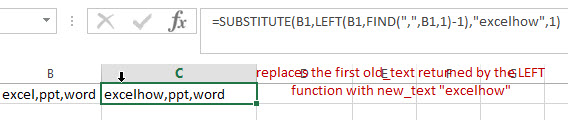This formula will replace the first old_text returned by the LEFT function with new_text “excelhow” in a text string in Cell B1.

If you want to replace all old_text with new_text, you just need to remove the third argument of the SUBSTITUTE function, just like the below:

=SUBSTITUTE(B1,LEFT(B1,FIND(“,”,B1,1)-1),”excelhow”)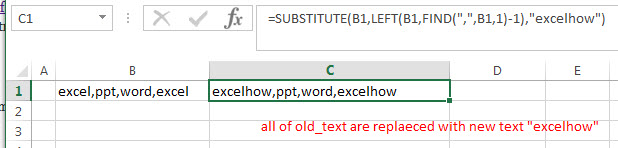You will see that all of old_text are replaeced with new text “excelhow” in Cell B1.

## Replace Text before a specific character using FIND&Select command

You can also use the Find and Replace command to replace text after a specified character, just refer to the following steps:

1# Click “HOME“->”Find&Select”->”Replace…”, then the window of the Find and Replace will appear.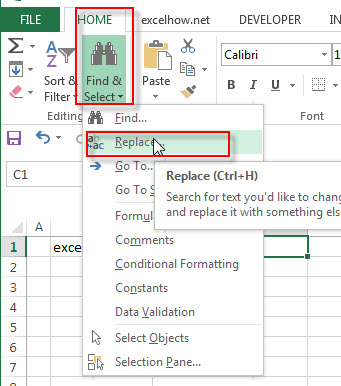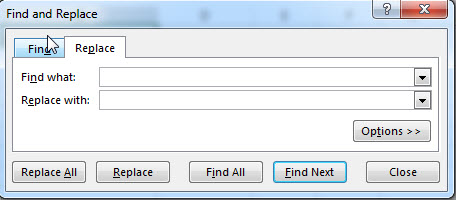2# click “Replace” Tab, then type e*, into the Find what: text box, and then type “excelhow” string in the Replace with: text box.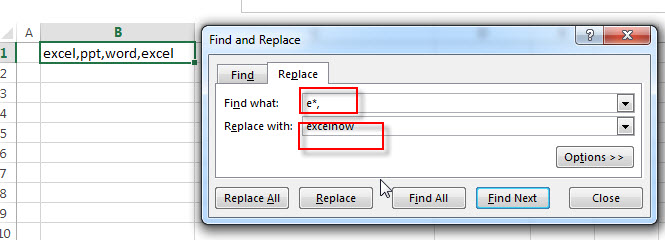3# click “Replace All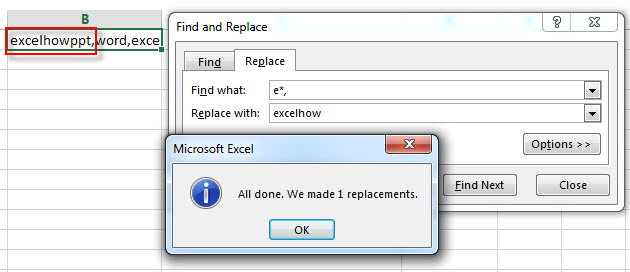You will see that all characters before the first comma character are replaced with “excelhow” string.

### Related Functions

• Excel LEFT function
The Excel LEFT function returns a substring (a specified number of the characters) from a text string, starting from the leftmost character.The LEFT function is a build-in function in Microsoft Excel and it is categorized as a Text Function.The syntax of the LEFT function is as below:= LEFT(text,[num_chars])…t)…
• Excel FIND function
The Excel FIND function returns the position of the first text string (sub string) within another text string.The syntax of the FIND function is as below:= FIND(find_text, within_text,[start_num])…
• Excel Substitute function
The Excel SUBSTITUTE function replaces a new text string for an old text string in a text string.The syntax of the SUBSTITUTE function is as below:= SUBSTITUTE  (text, old_text, new_text,[instance_num])….